## Programming Custom Light Sources and Uniform Deviates

Category: Product NewsOne of the most powerful features of OpticStudio is its open architecture, which allows motivated users to create custom sources to match the physical properties of their particular problem. This article explains how to program custom light sources and uniform deviates in Non-Sequential Mode.

Although OpticStudio comes with an impressive suite of light sources, many LED illumination profiles, radiant gaseous emitters, and laser fibers aren’t available from the menus. Indeed, one of the most useful visualization diagnostics - a simple 2D fan of rays - is not available.

Here, I will discuss a mathematical construct that appears to have confused many users: the inversion of uniform deviates as applied to source functions. This treatment rarely appears in the literature, so this note may be helpful for some.

Uniform deviates

Computers generate uniform deviates – uniformly/linearly distributed numbers within a range – when asked to produce random numbers. This is by convention rather than through any particular a priori mathematics, but is quite standard. This is also true regardless of whether one is using a system generated (i.e. Fortran callable or Mathcad supplied) random number, or one of many routines available in the literature which have some peculiar characteristics which are desired (fast, extremely uniform, repeatable or non-repeatable, etc.).  Typically, a random number generator (again by convention) will produce a result in the range (0,1], i.e. in the range from 0 to 1 including 0 and excluding 1 (this is not exclusive, some cases exist which include or exclude both 0 and 1).

Consider two such numbers, generated by your favorite random number generator, in the range (0,1].

Trivially, we often need to generate a physically relevant value, which is linearly related to a uniform deviate.  An excellent example is a source which can be located uniformly anywhere within a rectangular aperture. In this case, if the aperture is defined by the variable x on (0,x0], and likewise by y on (0,y0], we can easily use our two random deviates to properly select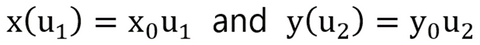Similarly, if we have a single angle, φ, which we want to vary uniformly on (0,2π]: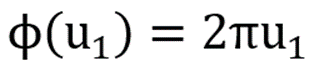Although these examples are trivial, they become less so if the mapping is non-uniform.  For example, we may have a source with a position uniformly distributed within a circular aperture instead of a rectangular aperture. Although the azumuthal angle is uniformly distributed in the range (0,2π], the radius is definitely not uniform in the range (0,r0].  If we did assume that the radius was linearly distributed in this range, we would generate a far higher density of source points close to the origin, and many fewer out at the periphery.

Instead, we want to ensure that the density of source points is uniform, regardless of how this is expressed.  Jumping ahead, we will find that this occurs if we use the following mapping: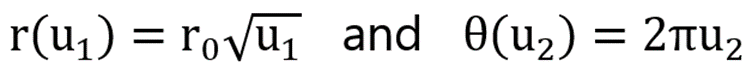Note that the formula for r(u1) satisfies the boundary conditions; it is bounded by r values of 0 and r0. Note also that it intuitively seems to be the right shape – it deforms the mapping correctly, moving density of sources away from 0 and out towards r0.

We can verify the correctness of this solution if we take note of the fact that du1 and du2 are both constant, since they are defined as being uniform in (0,1]. It is our desire that the density of sources in dA = dθ rdr is everywhere constant, as well as that the integral of dA over the range of admissible values yields the correct area of the circle: πr02 .

Since u1 and u2 are mutually independent, we can separate the problem like so: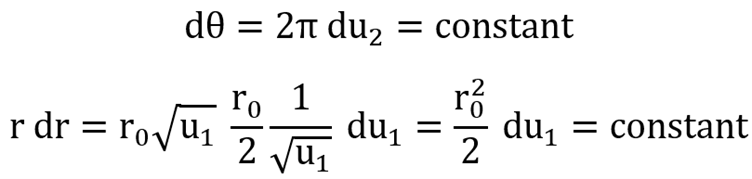Similarly, we can ensure that we are scaled correctly, by calculating the area of the circle two ways: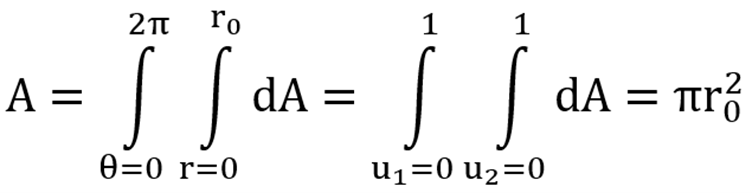Now let’s write down a procedure for getting to this sort of answer for any constraints. In addition, let’s generalize the answer to deal with those cases where we not only need to account for geometry, but also for a probability distribution which is not uniform.

First, we define a volume element, dr , which represents whatever dimensionality is relevant (area, volume, solid angle, etc). Second, we define a probability function P(r), which represents the physics of the profile we wish to generate.

We must first assure that the probability function is normalized, i.e. that the integral of the probability function over the relevant bounds of the volume adds up to unity.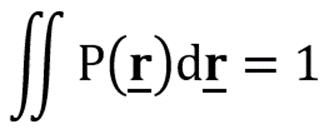Now, we re-apply the normalization integral over part of the bounds, such that the result is less than unity. By equating this sub-bounded integral to a uniform deviate, u1 , we find the proper value for r(u1) which properly represents the mapping from the uniform deviate u1 to the placement in space r . Let’s rework the last example this way. First, we have already separated out the azimuthal and radial components intuitively, so the volume element dr  is given by the one dimensional rdr. We want to produce a uniform source function over the area, so we define the probability function P(r) as a constant.

Normalization now sets the integral of P(r) over the circle equal to unity, thus we can now normalize the constant probability function as:(Note, this would be divided by 2π if we hadn’t separated out the azimuthal portion of the problem).

Finally, we now do the normalization integral where we bound the integral by r(u1). In effect, instead ofwe now haveThis step is the key to the solution – we are, in effect, taking the value u1, and scaling that to the appropriate fraction of the normalization integral.  The solution yields the radius at which we get this fraction of the unity normalization.

Solving this last gives us our desired result.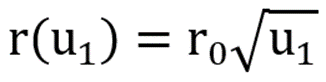Other examples

The technique above is universal.  Below are some examples which I have found handy in various source functions for clients.  For most of these, there is a second separable variable implied (usually azimuthal angle), which has a linear scaling with the independent u2 .

Uniform Illumination Angle – all solid angles

In this case, we want to generate an illumination angle which is uniformly distributed over  solid angle.  We separate variables into azimuthal and polar, and the relevant one-dimensional volume element is thus given by sinθ dθ. The probability function P(θ) is again constant, and normalization shows this constant as 1/2.

The mapping is found by solving the following integral.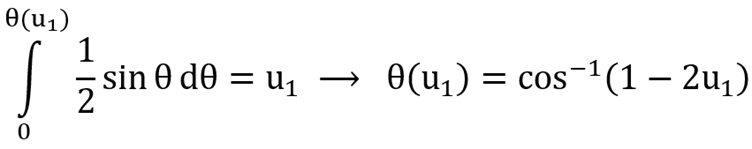Uniform Illumination Angle – limited solid angles

This case is identical to that above, except that we are only interested in generating rays with illumination angle limited to θ0 off the z-axis. The shape of the non-uniform deviate is the same, but the normalization now is performed over a more limited range, and instead of resulting in 1/2, it now normalizes to: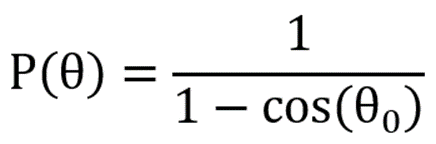The mapping is found by solving the integral.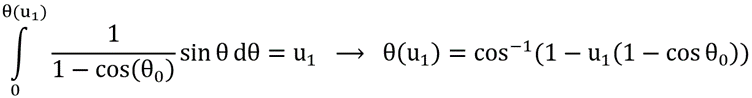Gaussian Distribution – Spherical Geometry

I have often found the need to model a diffuse source, such as a glowing plasma cloud. Usually, the relevant physics suggests that the source density is that of a radial Gaussian distribution and uniform angular distribution. For this, we generate the theta and phi of the position exactly as we did for uniform illumination angle over all solid angles. The radial distribution is a bit more complex. Our volume element is r2dr , and the probability function P(r) is now not constant, but instead has the shape exp[-(r/r0)2] (where r0 is the “1/e” radius).

We can do the normalization integral, this time bounded from zero to infinity, and solve for the leading normalization constant, thus:The mapping is found by solving the integral.Of course, this is not a particularly pleasant task, and solving for the function r(u1) requires some computational intensity.  Ultimately, a root finder and a Gaussian Error function generator are required, as the result reduces to the relation:which is not solvable analytically. Roots of this can be quickly obtained by Newton’s method, however.

Similar sources can be contrived in different geometries, or with deformed Gaussian distributions as constrained by external fields or other boundary conditions. A little more work can yield results where the cloud is of intermediate optical density – i.e. partially reabsorbing.

Although a little math is involved, these methods are highly efficient.  I have often seen people using the more intuitive method of generating candidate origins or angles from a larger space, and then throwing out candidates based on the probability functions; an iterative approach. The advantages may be small for some cases (e.g. square vs circular apertures of uniform density), but the advantages are tremendous when dealing with unbounded sources (such as exponentially diminishing sources).

Finally, experience shows that it is easy to make mistakes in the initial analytic modeling with these procedures. Always check your work with known benchmark cases!

Try Ansys Zemax OpticStudio for yourself, request a free trial today!

Author:
Jeff Casey
Rockfield Research Inc.